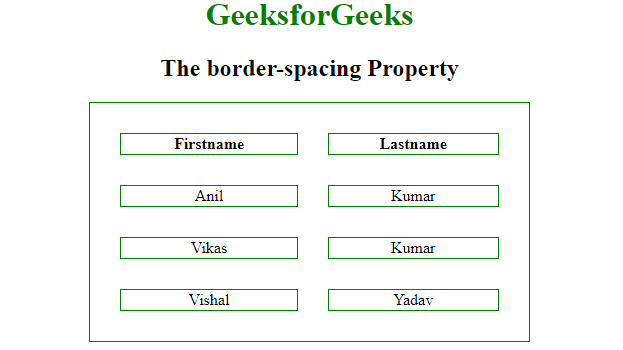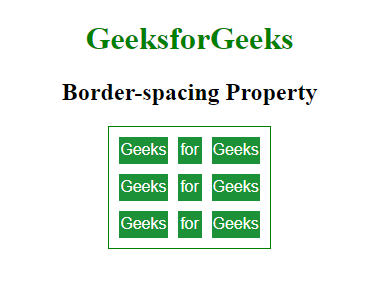# Which table property specifies the width that should appear between table cells in CSS ?

• Last Updated : 17 Jun, 2022

In this article, we will see how to specify the width that should appear between table cells. CSS provides the border-spacing property that can be used to set the distance between the borders of neighboring cells in the Table. This property works only when the border-collapse property is set to no-collapse separate.

Syntax:

`border-spacing: length|initial|inherit; `

Property values:

• length-length: This value is used to set the distance between the borders of adjacent cells. It does not allow negative values.
• initial: This value is used to sets the property to its default value.

Example: This example describes the border-spacing property by specifying the width that appears in between the table cell.

## HTML

 ```<``html``>`` ` `<``head``>``    ``<``title``>GeekforGeeks``    ``<``style``>``        ``table,``        ``th,``        ``td {``            ``border: 1px solid green;``            ``text-align: center;``        ``}``         ` `        ``.geeks {``            ``border-collapse: separate;``            ``background-color: none;``            ``border-spacing: 30px;``        ``}``         ` `        ``h1 {``            ``color: green;``        ``}``    `````` ` `<``body``>``    ``<``center``>``        ``<``h1``>GeeksforGeeks``        ``<``h2``> The border-spacing Property``         ` `        ``<``table` `style``=``"width:70%"` `class``=``"geeks"``>``            ``<``tr``>``                ``<``th``>Firstname``                ``<``th``>Lastname``                 ` `            ````            ``<``tr``>``                ``<``td``>Anil``                ``<``td``>Kumar``                 ` `            ````            ``<``tr``>``                ``<``td``>Vikas``                ``<``td``>Kumar``                 ` `            ````            ``<``tr``>``                ``<``td``>Vishal``                ``<``td``>Yadav``                 ` `            ````        ````    `````` ` `                `

Output:Example 2: This example describes the border-spacing property.

## HTML

 ```<``html``>`` ` `<``head``>``    ``<``title``>GeekforGeeks``    ``<``style``>``        ``table {``            ``border-spacing: 10px;``            ``border: 1px solid green;``        ``}``         ` `        ``td {``            ``width: 22px;``            ``height: 25px;``            ``background: #1d9138;``            ``color: white;``            ``text-align: center;``              ``font-family:sans-serif;``        ``}``    `````` ` `<``body``>``    ``<``center``>``        ``<``h1``>GeeksforGeeks``        ``<``h2``>Border-spacing Property``        ``<``table``>``            ``<``tr``>``                ``<``td``>Geeks``                ``<``td``>for``                ``<``td``>Geeks``            ````            ``<``tr``>``                ``<``td``>Geeks``                ``<``td``>for``                ``<``td``>Geeks``            ````            ``<``tr``>``                ``<``td``>Geeks``                ``<``td``>for``                ``<``td``>Geeks``            ````        ````    ```````

Output:My Personal Notes arrow_drop_up